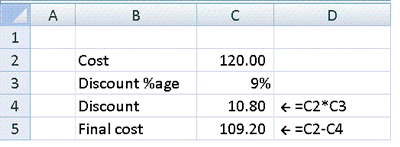# Calculate a discount of 9% on a purchase of 120

This is best approached in two stages.

First, calculate the percentage required using the technique described here - Finding a percentage of a number. Then subtract this amount from the original.

In the example below a 9% discount is applied to a purchase of 120.If you get a 9% discount on a purchase of 120, you only pay 109.20.

Try it yourself using the examples below...